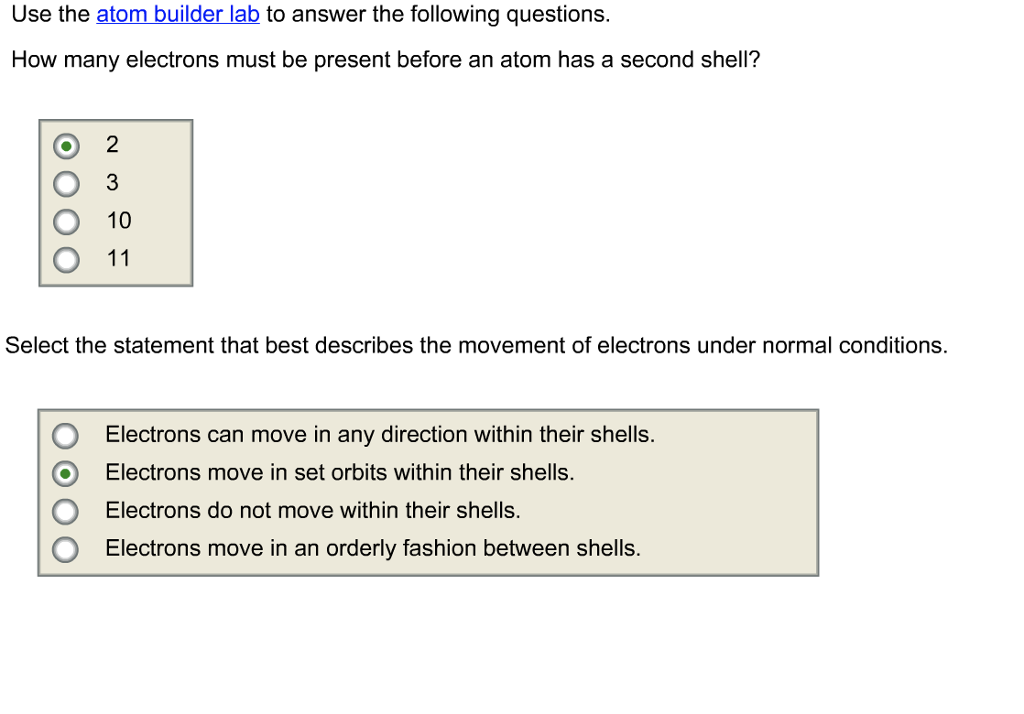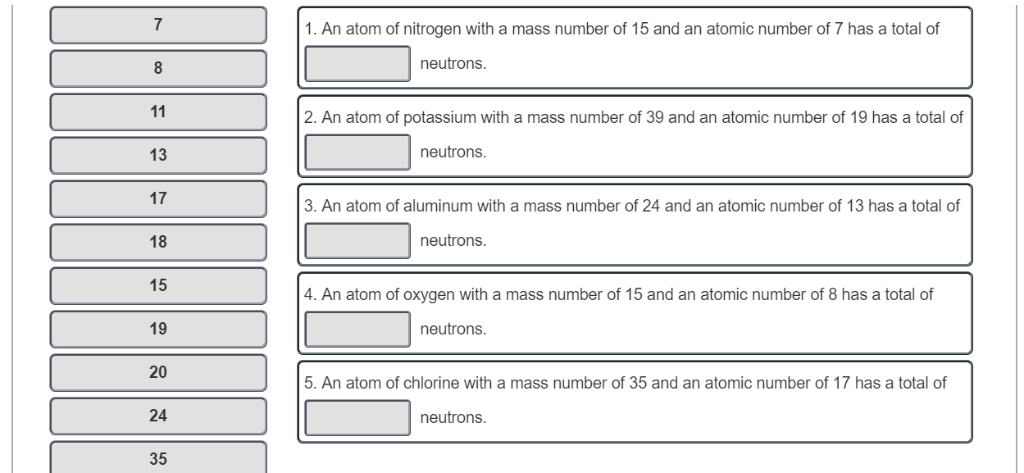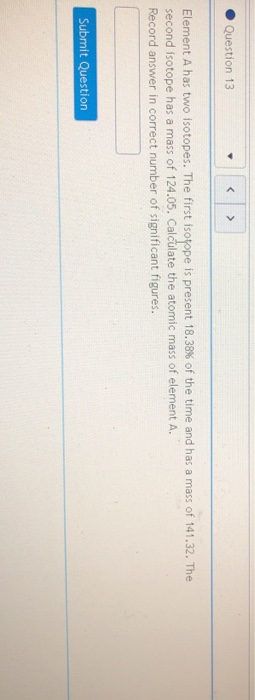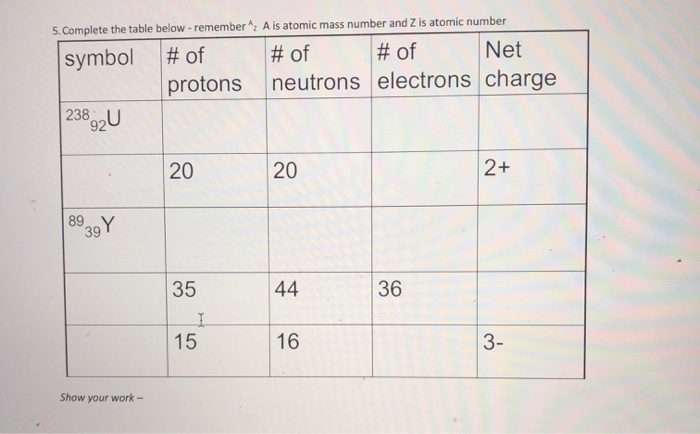Question

# How many electrons are present in an isotope of nitrogen that has a mass number of...

How many electrons are present in an isotope of nitrogen that has a mass number of 15 and no charge?

1- 15

2- 22

3- 8

4- 7

Number of electrons= atomic number- charge

For nitrogen atomic number = 7

Number of electrons= 7-0 = 7

#### Earn Coins

Coins can be redeemed for fabulous gifts.

Similar Homework Help Questions
• ### Determine how many electrons there are in total in your body and what is the total...

Determine how many electrons there are in total in your body and what is the total electrical charge of these electrons. Keep in mind that most of the atoms in your body have the same number of electrons, protons and neutrons. Consider the average composition of the human body of 75 kilograms mass as: 65% Oxygen, 19% Carbon, 10% Hydrogen, 3% Nitrogen, 2% Calcium and 1% Phosphorus. The atomic number of Oxygen is 8 and its atomic mass is 16...

• ### Use the atom builder lab to answer the following questions. How many electrons must be present...Use the atom builder lab to answer the following questions. How many electrons must be present before an atom has a second shell? 3 O 10 O 11 Select the statement that best describes the movement of electrons under normal conditions. OElectrons can move in any direction within their shells. O Electrons move in set orbits within their shells. Electrons do not move within their shells O Electrons move in an orderly fashion between shells. Largest mass number Smallest mass...

• ### 1. An atom of nitrogen with a mass number of 15 and an atomic number of...1. An atom of nitrogen with a mass number of 15 and an atomic number of 7 has a total of neutrons. 2. An atom of potassium with a mass number of 39 and an atomic number of 19 has a total of neutrons 13 17 18 15 19 20 24 35 3. An atom of aluminum with a mass number of 24 and an atomic number of 13 has a total of neutrons. 4. An atom of oxygen with...

• ### The most abundant isotope of lead is 20882Pb. Part A Part complete How many protons are...

The most abundant isotope of lead is 20882Pb. Part A Part complete How many protons are in the atom? Express your answer as an integer. Np = 82 Previous Answers Correct Part B Part complete How many neutrons are in the atom? Express your answer as an integer. Nn = 126 Previous Answers Correct Part C Part complete How many electrons are in the atom? Express your answer as an integer. Ne = 82 Previous Answers Correct Part D What...

• ### 1- an atom has the following chemical symbol: 114Cd a- how many protons does this atom...

1- an atom has the following chemical symbol: 114Cd a- how many protons does this atom have? b- how many neutrons does this atom have? c- how many electrons does this atom have? 2- an ion has 33 protons, 41 neutrons, and 36 electrons a- what is the mass number of this atom? b- what is the symbol for this ion? enter both mass and the atomic number 3- which of these ions has the same number of electrons as...

• ### Identify the missing information for each neutral isotope. A Se atom has a mass number of...

Identify the missing information for each neutral isotope. A Se atom has a mass number of 79. Determine the number of neutrons, protons, and electrons in this neutral isotope. number of neutrons: number of protons: number of electrons: A neutral isotope has a mass number of 65 and 36 neutrons. Identify the element symbol of this isotope and determine the number of protons and electrons. element symbol: number of protons: number of electrons: A neutral isotope has 49 neutrons and...

• ### 1. How many moles are present in 1.51 x 1023 molecules of HCl? A. 9.12 mol...

1. How many moles are present in 1.51 x 1023 molecules of HCl? A. 9.12 mol B. 0.251 mol C. 3.99 mol D. 146 mol 2. Ions can be formed from atoms by losing or gaining electrons. Select the alternative that states the correct number of protons, neutrons, and electrons in Cu2+ with mass number 63. A. 29 protons, 34 neutrons, 31 electrons B. 29 protons, 34 neutrons, 27 electrons C. 27 protons, 29 neutrons, 29 electrons D. 29 protons,...

• ### 1. How many GRAMS of nitrogen are present in 1.55 moles of dinitrogen tetrafluoride, N2F4?   _______grams...

1. How many GRAMS of nitrogen are present in 1.55 moles of dinitrogen tetrafluoride, N2F4?   _______grams 2. How many MOLES of fluorine are present in 1.08 grams of dinitrogen tetrafluoride?   _________moles 3.The percent by mass of copper(I) in Cu2SO3 is __________%. 4. Calculate the percentage composition for SF4. Mass percentage of sulfur = _________% Mass percentage of fluorine = _________%

• ### Question 13 < > Element A has two isotopes. The first isotope is present 18.38% of...Question 13 < > Element A has two isotopes. The first isotope is present 18.38% of the time and has a mass of 141.32. The second isotope has a mass of 124.05. Calculate the atomic mass of element A. Record answer in correct number of significant figures. Submit Question

• ### A is atomic mass number and Z is atomic number 5. Complete the table below -remember Net #of neutrons electrons ch...A is atomic mass number and Z is atomic number 5. Complete the table below -remember Net #of neutrons electrons charge #of symbol #of protons 238a2U 20 2+ 20 899Y 39 36 35 44 15 16 3- Show your work- LO 24Mg, 25Mg and 26Mg. 6. Calculate the elemental atomic mass of Mg if the naturally occurring isotopes Their masses and abundances are as follows: Isotope Atomic 24-Mg 25-Mg 26-Mg are Mass Isotopic 23.98504 amu Abundance 78.70% 24.98584 amu 10.13%...